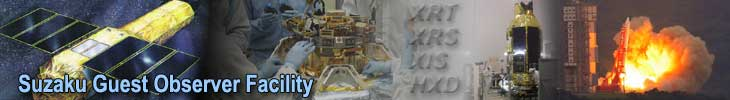## XISCONTAMICALC

Xiscontamicalc calculates the thickness and the X-ray transmission of the XIS OBF contaminants as a function of X-ray energy, time, detector ID, and location on the detector. The function is also used internally in xissimarfgen.

The stand-alone tool, xiscontamicalc is convenient

1. when a user wishes to use an ARF that does not include the effects of the contaminant, instead including it in the spectral model; and
2. for modifying an existing ARF file with the contaminant transmission.

The parameters of the spatially dependent growth of the contaminant for each XIS detector are available in the Suzaku CALDB files (ae_xiN_contami_YYYYMMDD.fits). Xiscontamicalc reads the appropriate file for that detector and prints out the carbon column density at the user-specified location and time. It also outputs the X-ray transmission for the user-specified energy. The current calibration file assumes a C/O ratio of 6:1 by number.

### Estimating the degree of contamination

First, a user needs to find the detector coordinates of the region of interest. We here show two ways of obtaining the position.

1. Make an image in the detector coordinate with xselect or ds9 and find the source position by eye. In xselect, type set xyname detx dety before making an image. In ds9, choose DETX/DETY bin column in the binning parameter option in the Bin menu (click "or center of data" to display the whole image).
2. Use the Suzaku tool aecoordcalc. For example, one can obtain the detector coordinate of a point source at (R.A., Dec) = (161.043071, -59.719836) in observation 100012010 as follows:
• aecoordcalc instrume=XIS1 coord=J2000 alpha=161.043071 delta=-59.719836 output=FULL attitude==ae102002010.att pointing=KEY infile=ae102002010xi1_0_3x3n069b_cl.evt t=2005-08-29T12:00:00
• The output (captured in this file) shows (DETX, DETY) = (898.0, 491.1).

Using the above result, one can then estimate the degree of contamination on XIS1 at 2006-08-29T12:00:00 and the transmission at 1 keV as follows:

```> xiscontamicalc instrume=XIS1 \
date_obs=2007-06-14T02:00:00 energy=1.0 detx=770.5 dety=704.5
arffile=none scale_contaminant=no
xiscontamicalc version 2006-11-26
Written by Y.ISHISAKI (TMU)
Built on ANL HEADAS converter 1.80 for ANL version 1.80
ngp=522, t0=177242000.0, t1=492342800.0, nen=7900, e0=0.201, e1=15.999

TELESCOP = 'SUZAKU'
INSTRUME = 'XIS1'
CONTAMIFILE = '/FTP/caldb/data/suzaku/xis/bcf/ae_xi1_contami_20061016.fits' (CALDB)
LEAPFILE = '/FTP/caldb/data/gen/bcf/leapsec_010905.fits' (CALDB)
DATE-OBS = '2007-06-14T02:00:00' ( TIME = 235101601.000000 )
DAYS = 669.671 [dy] after 2005-08-13T09:53:20
ENERGY = 1.000 [keV]
R_OFFSET = 5.586 [arcmin] at ( DETX , DETY ) = ( 770.5 , 704.5 ) [pixel]
CARBON = 3.749518 [10**18 cm**-2]
OXYGEN = 0.624920 [10**18 cm**-2]
TRANSMIS = 7.822524e-01
```

Here, the column densities of Carbon and Oxygen are listed in the "CARBON" and "OXYGEN" rows, respectively. In a spectral fit with xspec, one simply multiplies the assumed source spectrum by the varabs model with the carbon and oxygen column densities given above and column densities of the other elements set to zero. This should be done using an ARF that does not include the contaminant transmission (see below). The "TRANSMIS" row shows the transmission of X-rays at 1 keV.

### Modifying an existing ARF file with the contaminant transmission

a). Multiply the effective areas in the arf file ae_xi1_xisnom6_contami1.arf with the contamination transmission at date_obs=2006-03-01T00:00:00, (DETX, DETY)=(310, 512.5). The result does not depends on values in the energy option.

```% xiscontamicalc instrume=XIS1 date_obs=2006-03-01T00:00:00 energy=1.0 \
detx=310 dety=512.5 arffile=ae_xi1_xisnom6_contami1.arf scale_contaminant=no
```

b). Modifying an ARF to remove the effect of the contaminant transmission. In the following command, the energy, detx/dety date_obs options are ignored.

```% xiscontamicalc instrume=XIS1 date_obs=2006-03-01T00:00:00 energy=1.0 \
detx=310 dety=512.5 arffile=ae_xi1_xisnom6_contami3.arf scale_contaminant=yes \
scale_previous=yes scale_factor=0.0
```

If you have any questions concerning Suzaku, visit the Feedback form.

 A service of the Astrophysics Science Division (ASD) at NASA/ GSFC Suzaku Project Scientist: Dr. Robert Petre Responsible NASA Official: Phil Newman